Ex 4.5

Chapter 4 Class 12 Determinants
Serial order wise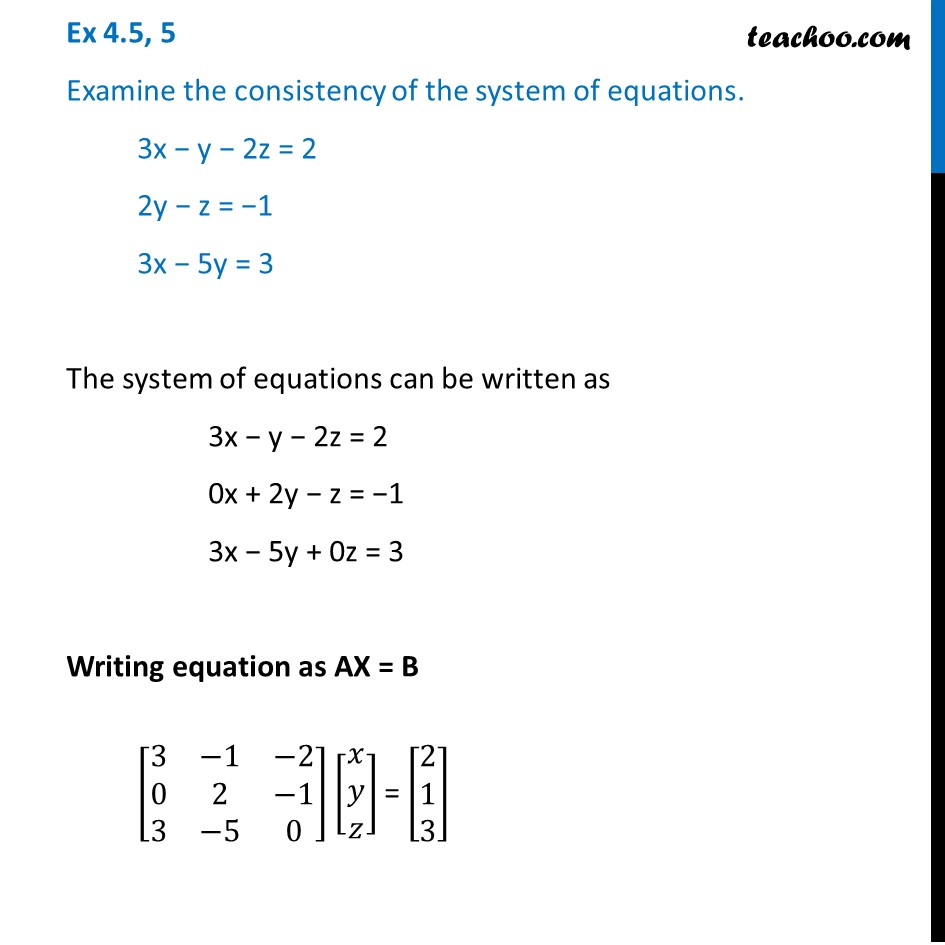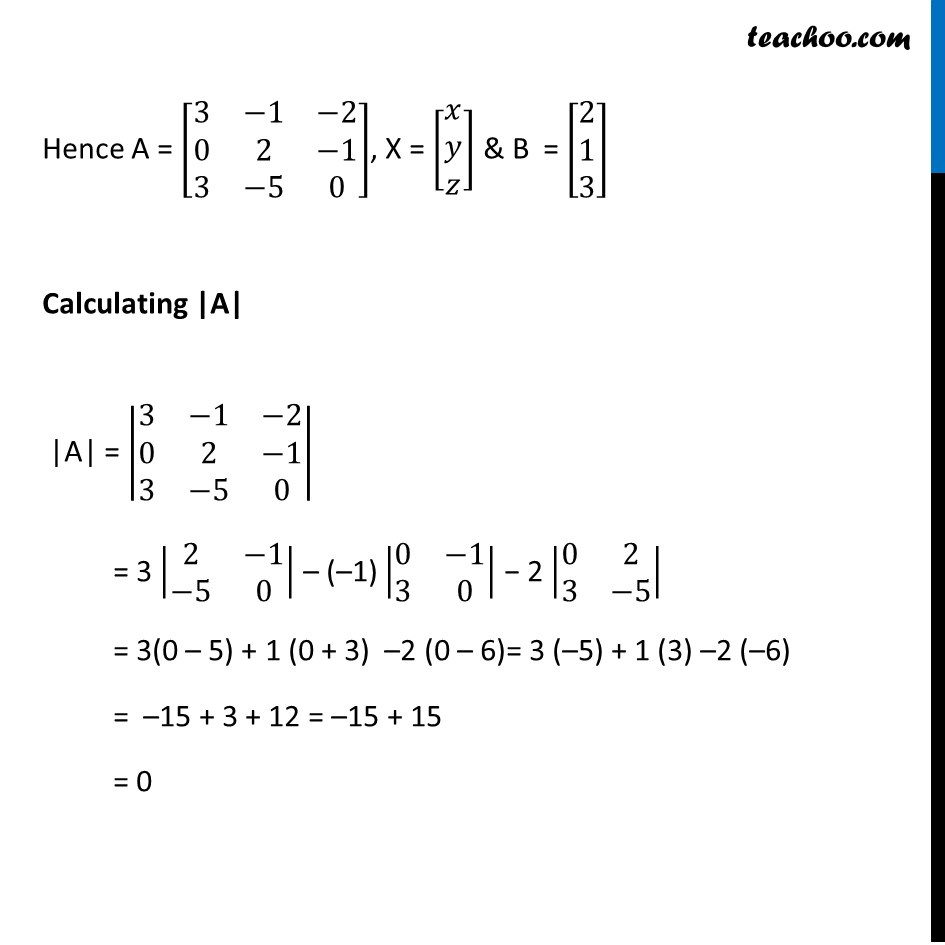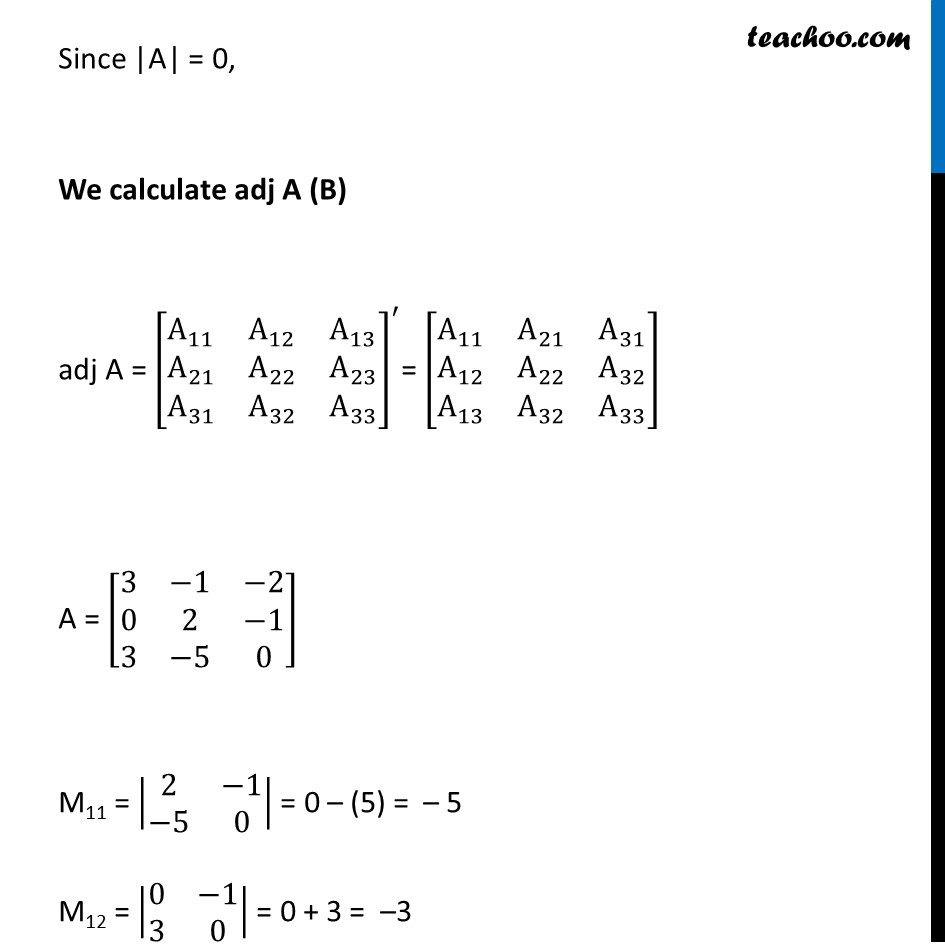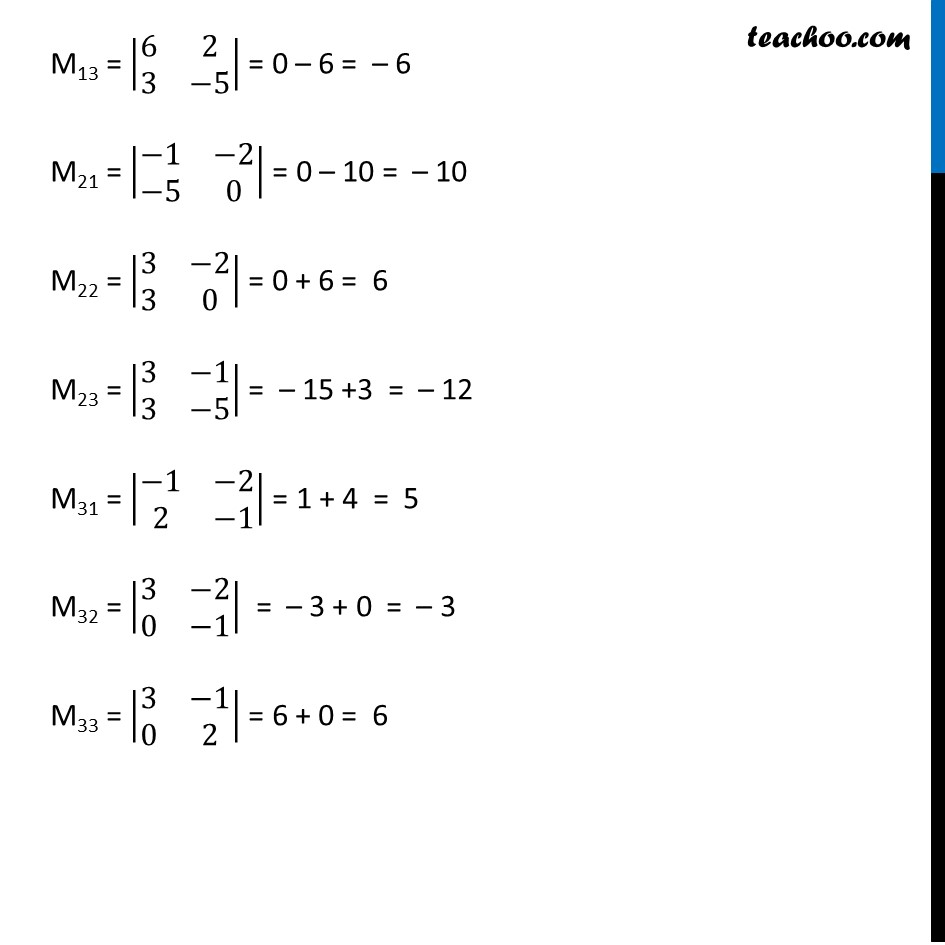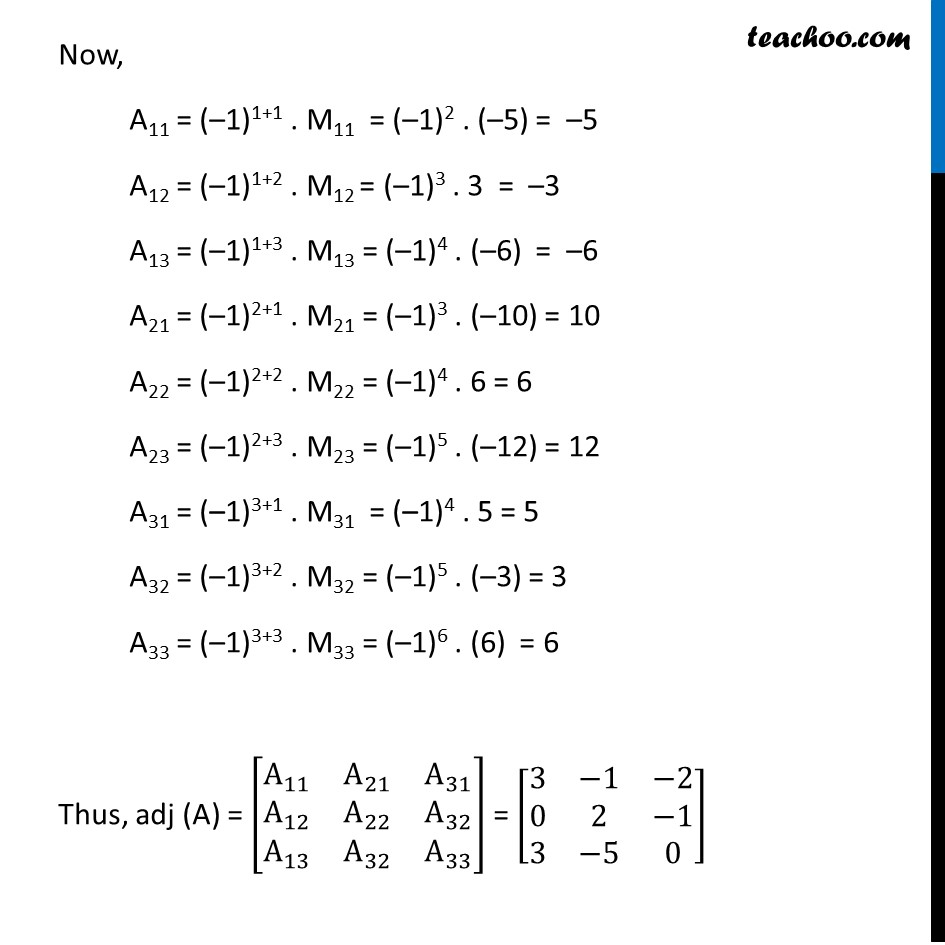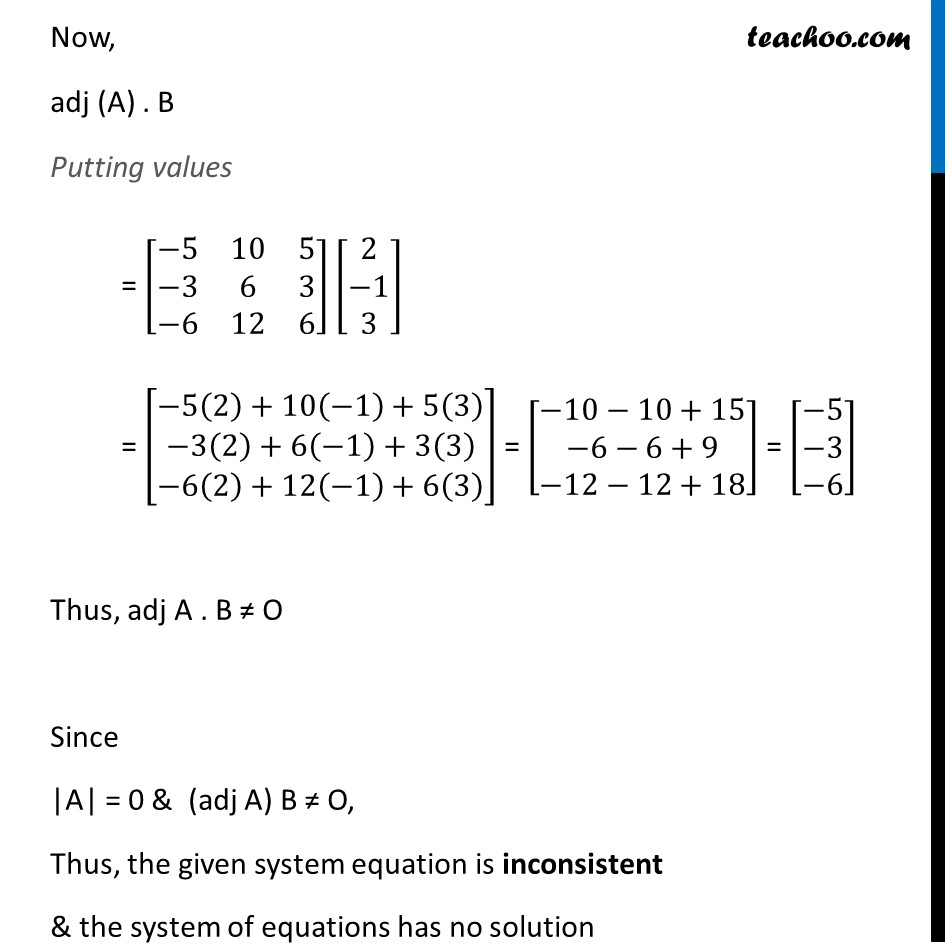Learn in your speed, with individual attention - Teachoo Maths 1-on-1 Class

### Transcript

Ex 4.5, 5 Examine the consistency of the system of equations. 3x − y − 2z = 2 2y − z = −1 3x − 5y = 3 The system of equations can be written as 3x − y − 2z = 2 0x + 2y − z = −1 3x − 5y + 0z = 3 Writing equation as AX = B [■8(3&−1&−2@0&2&−1@3&−5&0)] [■8(𝑥@𝑦@𝑧)] = [■8(2@1@3)] Hence A = [■8(3&−1&−2@0&2&−1@3&−5&0)], X = [■8(𝑥@𝑦@𝑧)] & B = [■8(2@1@3)] Calculating |A| |A| = |■8(3&−1&−2@0&2&−1@3&−5&0)| = 3 |■8(2&−1@−5&0)| – (–1) |■8(0&−1@3&0)| − 2 |■8(0&2@3&−5)| = 3(0 – 5) + 1 (0 + 3) –2 (0 – 6)= 3 (–5) + 1 (3) –2 (–6) = –15 + 3 + 12 = –15 + 15 = 0 Since |A| = 0, We calculate adj A (B) adj A = [■8(A_11&A_12&A_13@A_21&A_22&A_23@A_31&A_32&A_33 )]^′= [■8(A_11&A_21&A_31@A_12&A_22&A_32@A_13&A_32&A_33 )] A = [■8(3&−1&−2@0&2&−1@3&−5&0)] M11 = |■8(2&−1@−5&0)| = 0 – (5) = – 5 M12 = |■8(0&−1@3&0)| = 0 + 3 = –3 M13 = |■8(6&2@3&−5)| = 0 – 6 = – 6 M21 = |■8(−1&−2@−5&0)| = 0 – 10 = – 10 M22 = |■8(3&−2@3&0)| = 0 + 6 = 6 M23 = |■8(3&−1@3&−5)| = – 15 +3 = – 12 M31 = |■8(−1&−2@2&−1)| = 1 + 4 = 5 M32 = |■8(3&−2@0&−1)| = – 3 + 0 = – 3 M33 = |■8(3&−1@0&2)| = 6 + 0 = 6 Now, A11 = (–1)1+1 . M11 = (–1)2 . (–5) = –5 A12 = (–1)1+2 . M12 = (–1)3 . 3 = –3 A13 = (–1)1+3 . M13 = (–1)4 . (–6) = –6 A21 = (–1)2+1 . M21 = (–1)3 . (–10) = 10 A22 = (–1)2+2 . M22 = (–1)4 . 6 = 6 A23 = (–1)2+3 . M23 = (–1)5 . (–12) = 12 A31 = (–1)3+1 . M31 = (–1)4 . 5 = 5 A32 = (–1)3+2 . M32 = (–1)5 . (–3) = 3 A33 = (–1)3+3 . M33 = (–1)6 . (6) = 6 Thus, adj (A) = [■8(A_11&A_21&A_31@A_12&A_22&A_32@A_13&A_32&A_33 )] = [■8(3&−1&−2@0&2&−1@3&−5&0)] Now, adj (A) . B Putting values = [■8(−5&10&5@−3&6&3@−6&12&6)] [■8(2@−1@3)] = [■8(−5(2)+10(−1)+5(3)@−3(2)+6(−1)+3(3)@−6(2)+12(−1)+6(3) )] = [■8(−10−10+15@−6−6+9@−12−12+18)] = [■8(−5@−3@−6)] Thus, adj A . B ≠ O Since |A| = 0 & (adj A) B ≠ O, Thus, the given system equation is inconsistent & the system of equations has no solution